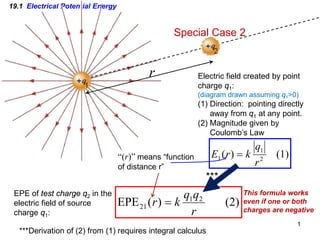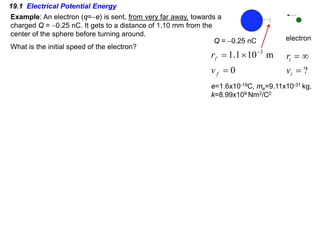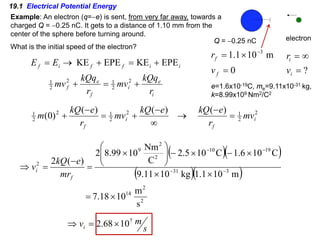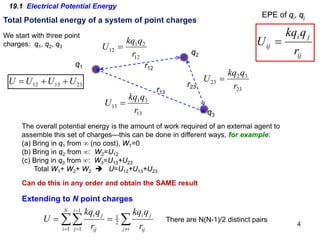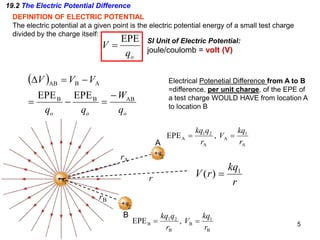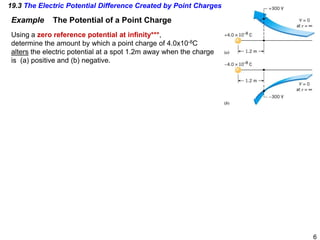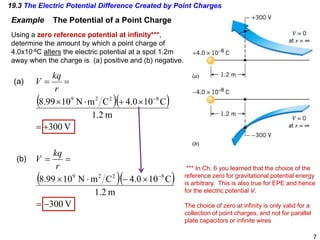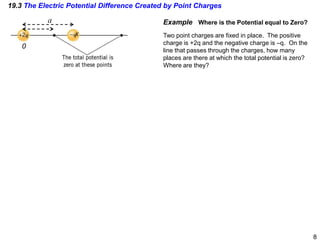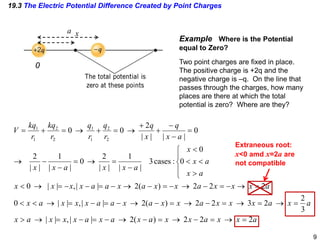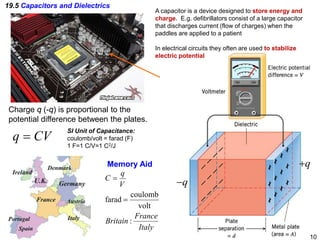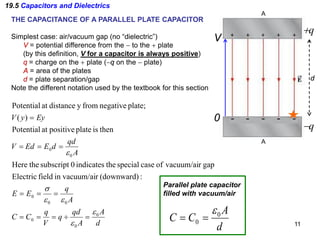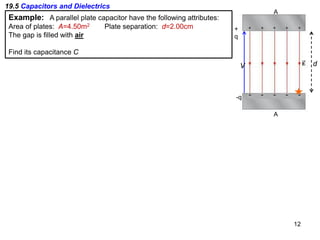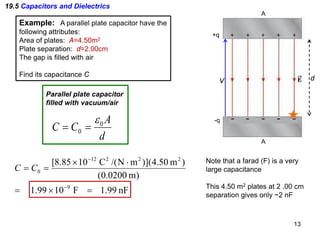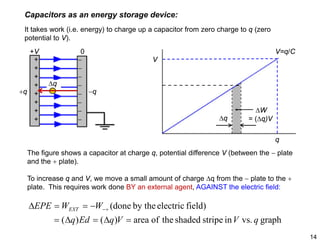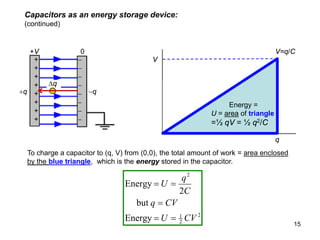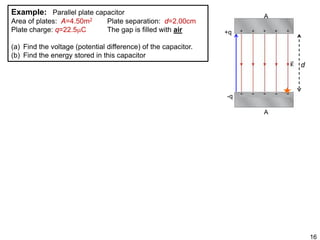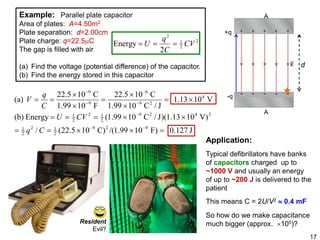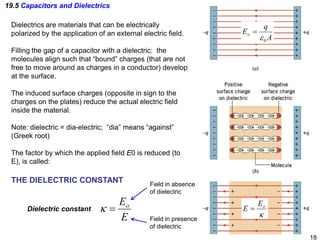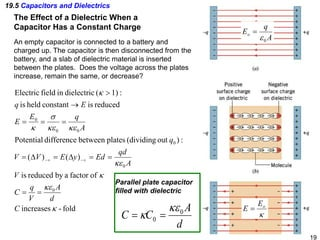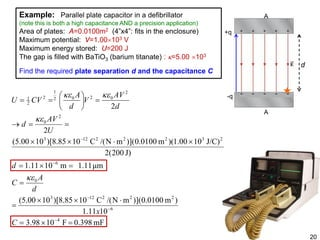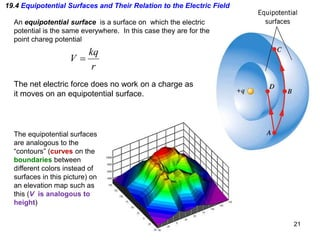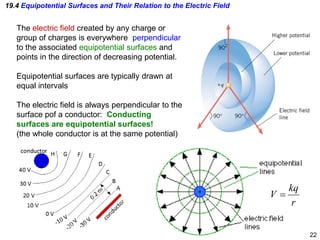1 of 22

### jan25.pdf

1. 1 19.1 Electrical Potential Energy r Special Case 2 EPE of test charge q2 in the electric field of source charge q1: Electric field created by point charge q1: (diagram drawn assuming q1>0) (1) Direction: pointing directly away from q1 at any point. (2) Magnitude given by Coulomb’s Law (1) ) ( 2 1 1 r q k r E = (2) ) ( EPE 2 1 21 r q q k r = ***Derivation of (2) from (1) requires integral calculus This formula works even if one or both charges are negative *** “(r)” means “function of distance r”
2. 19.1 Electrical Potential Energy Example: An electron (q=−e) is sent, from very far away, towards a charged Q = −0.25 nC. It gets to a distance of 1.10 mm from the center of the sphere before turning around. What is the initial speed of the electron? Q = −0.25 nC electron ? = ∞ = i i v r 0 m 10 1 . 1 3 = × = − f f v r e=1.6x10-19C, me=9.11x10-31 kg, k=8.99x109 Nm2/C2
3. 19.1 Electrical Potential Energy Example: An electron (q=−e) is sent, from very far away, towards a charged Q = −0.25 nC. It gets to a distance of 1.10 mm from the center of the sphere before turning around. What is the initial speed of the electron? Q = −0.25 nC electron ? = ∞ = i i v r 0 m 10 1 . 1 3 = × = − f f v r i e i f e f i i f f i f r kQq mv r kQq mv E E + = + + = + → = 2 2 1 2 2 1 EPE KE EPE KE 2 2 1 2 2 1 2 2 1 ) ( ) ( ) ( ) 0 ( i f i f mv r e kQ e kQ mv r e kQ m = − → ∞ − + = − + ( )( ) ( )( ) 2 2 14 3 31 19 10 2 2 9 2 s m 10 18 . 7 m 10 1 . 1 kg 10 11 . 9 C 10 6 . 1 C 10 5 . 2 C Nm 10 99 . 8 2 ) ( 2 × = × × × − × −         × = − = ⇒ − − − − f i mr e kQ v s m vi 7 10 68 . 2 × = ⇒ e=1.6x10-19C, me=9.11x10-31 kg, k=8.99x109 Nm2/C2
4. The overall potential energy is the amount of work required of an external agent to assemble this set of charges—this can be done in different ways, for example: (a) Bring in q1 from ∞ (no cost), W1=0 (b) Bring in q2 from ∞: W2=U12 (c) Bring in q3 from ∞: W3=U13+U23 Total W1+ W2+ W2  U=U12+U13+U23 Can do this in any order and obtain the SAME result 4 Total Potential energy of a system of point charges q2 q3 q1 r12 r13 r23 ij j i ij r q kq U = 12 2 1 12 r q kq U = 13 3 1 13 r q kq U = 23 3 2 23 r q kq U = 23 13 12 U U U U + + = ∑ ∑∑ ≠ = − = = = i j ij j i N i i j ij j i r q kq r q kq U 2 1 1 1 1 There are N(N-1)/2 distinct pairs Extending to N point charges 19.1 Electrical Potential Energy We start with three point charges: q1, q2, q3 EPE of qi, qj
5. 19.2 The Electric Potential Difference DEFINITION OF ELECTRIC POTENTIAL The electric potential at a given point is the electric potential energy of a small test charge divided by the charge itself: o q V EPE = SI Unit of Electric Potential: joule/coulomb = volt (V) ( ) o o o q W q q V V V AB B B A B AB EPE EPE − = − = − = ∆ 5 Electrical Potenetial Difference from A to B =difference, per unit charge, of the EPE of a test charge WOULD HAVE from location A to location B rA rB A B A 1 A A 2 1 A , EPE r kq V r q kq = = B 1 B B 2 1 B , EPE r kq V r q kq = = r kq r V 1 ) ( = r
6. 19.3 The Electric Potential Difference Created by Point Charges Example The Potential of a Point Charge Using a zero reference potential at infinity***, determine the amount by which a point charge of 4.0x10-8C alters the electric potential at a spot 1.2m away when the charge is (a) positive and (b) negative. 6
7. 19.3 The Electric Potential Difference Created by Point Charges Example The Potential of a Point Charge Using a zero reference potential at infinity***, determine the amount by which a point charge of 4.0x10-8C alters the electric potential at a spot 1.2m away when the charge is (a) positive and (b) negative. 7 ( )( ) V 300 m 2 . 1 C 10 0 . 4 C m N 10 99 . 8 8 2 2 9 + = × + ⋅ × = = − r kq V (a) ( )( ) V 300 m 2 . 1 C 10 0 . 4 C m N 10 99 . 8 8 2 2 9 − = × − ⋅ × = = − r kq V (b) *** In Ch. 6 you learned that the choice of the reference zero for gravitational potential energy is arbitrary. This is also true for EPE and hence for the electric potential V. The choice of zero at infinity is only valid for a collection of point charges, and not for parallel plate capacitors or infinite wires
8. 19.3 The Electric Potential Difference Created by Point Charges Example Where is the Potential equal to Zero? Two point charges are fixed in place. The positive charge is +2q and the negative charge is –q. On the line that passes through the charges, how many places are there at which the total potential is zero? Where are they? 8 a 0 x
9. 19.3 The Electric Potential Difference Created by Point Charges Example Where is the Potential equal to Zero? Two point charges are fixed in place. The positive charge is +2q and the negative charge is –q. On the line that passes through the charges, how many places are there at which the total potential is zero? Where are they? 9 a 0 x 2 2 2 ) ( 2 | | , | | 3 2 2 3 2 2 ) ( 2 | | , | | 0 2 2 2 ) ( 2 | | , | | 0 0 0 : cases 3 | | 1 | | 2 0 | | 1 | | 2 0 | | | | 2 0 0 2 2 1 1 2 2 1 1 a x x a x x a x a x a x x x a x a x a x x x a x x a x a a x x x a x a x x x a x x a x a a x x x x a x a x x a x x a x x a x q x q r q r q r kq r kq V = → = − → = − → − = − = → > = → = → = − → = − → − = − = → < < = → − = − → − = − → − = − − = → <      > < < < − = → = − − → = − − + + → = + → = + = Extraneous root: x<0 amd x=2a are not compatible
10. +q −q 19.5 Capacitors and Dielectrics Charge q (-q) is proportional to the potential difference between the plates. Italy France Britain V q C : volt coulomb farad = = SI Unit of Capacitance: coulomb/volt = farad (F) 1 F=1 C/V=1 C2/J 10 CV q = Memory Aid A capacitor is a device designed to store energy and charge. E.g. defibrillators consist of a large capacitor that discharges current (flow of charges) when the paddles are applied to a patient In electrical circuits they often are used to stabilize electric potential
11. 19.5 Capacitors and Dielectrics THE CAPACITANCE OF A PARALLEL PLATE CAPACITOR Simplest case: air/vacuum gap (no “dielectric”) V = potential difference from the − to the + plate (by this definition, V for a capacitor is always positive) q = charge on the + plate (−q on the − plate) A = area of the plates d = plate separation/gap Note the different notation used by the textbook for this section 11 +q A A d V d A A qd q V q C C A q E E A qd d E Ed V Ey y V 0 0 0 0 0 0 0 0 : (downward) r vacuum/ai in field Electric gap r vacuum/ai of case special the indicates 0 subscript the Here then is plate positive at Potential ) ( plate; negative from y distance at Potential ε ε ε ε σ ε = ÷ = = = = = = = = = = Parallel plate capacitor filled with vacuum/air d A C C 0 0 ε = = −q 0
12. 12 19.5 Capacitors and Dielectrics Example: A parallel plate capacitor have the following attributes: Area of plates: A=4.50m2 Plate separation: d=2.00cm The gap is filled with air Find its capacitance C + q -q A A d V
13. 13 19.5 Capacitors and Dielectrics Example: A parallel plate capacitor have the following attributes: Area of plates: A=4.50m2 Plate separation: d=2.00cm The gap is filled with air Find its capacitance C Parallel plate capacitor filled with vacuum/air d A C C 0 0 ε = = nF 99 . 1 F 10 99 . 1 ) m 0200 . 0 ( ) m 50 . 4 )]( m N /( C 10 85 . 8 [ 9 2 2 2 12 0 = × = ⋅ × = = − − C C Note that a farad (F) is a very large capacitance This 4.50 m2 plates at 2 .00 cm separation gives only ~2 nF +q -q A A d V
14. 14 Capacitors as an energy storage device: It takes work (i.e. energy) to charge up a capacitor from zero charge to q (zero potential to V). The figure shows a capacitor at charge q, potential difference V (between the − plate and the + plate). To increase q and V, we move a small amount of charge ∆q from the − plate to the + plate. This requires work done BY an external agent, AGAINST the electric field: V q ∆q ∆W = (∆q)V V=q/C +V 0 + + + + + + + + − − − − − − − − ∆q +q −q graph vs. in stripe shaded the of area ) ( ) ( field) electric by the (done q V V q Ed q W W EPE EXT = ∆ = ∆ = − = = ∆ + −
15. 15 Capacitors as an energy storage device: (continued) To charge a capacitor to (q, V) from (0,0), the total amount of work = area enclosed by the blue triangle, which is the energy stored in the capacitor. V q ∆q ∆W = (∆q)V V=q/C +V 0 + + + + + + + + − − − − − − − − ∆q +q −q 2 2 1 2 Energy but 2 Energy CV U CV q C q U = = = = = Energy = U = area of triangle =½ qV = ½ q2/C
16. 16 +q -q A A d Example: Parallel plate capacitor Area of plates: A=4.50m2 Plate separation: d=2.00cm Plate charge: q=22.5µC The gap is filled with air (a) Find the voltage (potential difference) of the capacitor. (b) Find the energy stored in this capacitor
17. 17 +q -q A A d Example: Parallel plate capacitor Area of plates: A=4.50m2 Plate separation: d=2.00cm Plate charge: q=22.5µC The gap is filled with air (a) Find the voltage (potential difference) of the capacitor. (b) Find the energy stored in this capacitor 2 2 1 2 2 Energy CV C q U = = = J 127 . 0 ) F 10 99 . 1 /( C) 10 22.5 ( / V) 10 13 . 1 )( J / C 10 99 . 1 ( Energy (b) V 10 13 . 1 J / C 10 99 . 1 C 10 22.5 F 10 99 . 1 C 10 22.5 (a) 9 2 9 2 1 2 2 1 2 4 2 9 2 1 2 2 1 4 2 9 6 9 9 = × × = = × × = = = × = × × = × × = = − − − − − − − C q CV U C q V Application: Typical defibrillators have banks of capacitors charged up to ~1000 V and usually an energy of up to ~200 J is delivered to the patient This means C = 2U/V2 ≈ 0.4 mF So how do we make capacitance much bigger (approx. ×105)? Resident Evil?
18. 19.5 Capacitors and Dielectrics Dielectric constant E Eo = κ 18 Dielectrics are materials that can be electrically polarized by the application of an external electric field. Filling the gap of a capacitor with a dielectric: the molecules align such that “bound” charges (that are not free to move around as charges in a conductor) develop at the surface. The induced surface charges (opposite in sign to the charges on the plates) reduce the actual electric field inside the material. Note: dielectric = dia-electric; “dia” means “against” (Greek root) The factor by which the applied field E0 is reduced (to E), is called: THE DIELECTRIC CONSTANT Field in absence of dielectric Field in presence of dielectric κ o E E = A q Eo 0 ε =
19. 19.5 Capacitors and Dielectrics The Effect of a Dielectric When a Capacitor Has a Constant Charge An empty capacitor is connected to a battery and charged up. The capacitor is then disconnected from the battery, and a slab of dielectric material is inserted between the plates. Does the voltage across the plates increase, remain the same, or decrease? 19 fold - increases of factor a by reduced is ) ( ) ( : ) out (dividing plates between difference Potential reduced is constant held is : 1) ( dielectric in field Electric 0 0 0 0 0 0 κ κε κ κε κε κε σ κ κ C d A V q C V A qd Ed y E V V q A q E E E q = = = = ∆ = ∆ = = = = → > + − + − A q Eo 0 ε = κ o E E = Parallel plate capacitor filled with dielectric d A C C 0 0 κε κ = =
20. 20 +q -q A A d Example: Parallel plate capacitor in a defibrillator (note this is both a high capacitance AND a precision application) Area of plates: A=0.0100m2 (4”x4”: fits in the enclosure) Maximum potential: V=1.00×103 V Maximum energy stored: U=200 J The gap is filled with BaTiO3 (barium titanate) : κ=5.00 ×103 Find the required plate separation d and the capacitance C mF 0.398 F 10 98 . 3 10 11 . 1 ) m 0100 . 0 )]( m N /( C 10 85 . 8 )[ 10 00 . 5 ( μm 11 . 1 m 10 11 . 1 J) 200 ( 2 J/C) 10 00 . 1 )( m 0100 . 0 )]( m N /( C 10 85 . 8 )[ 10 00 . 5 ( 2 2 4 6 2 2 2 12 3 0 6 2 3 2 2 2 12 3 2 0 2 0 2 0 2 1 2 2 1 = × = ⋅ × × = = = × = × ⋅ × × = = → =       = = − − − − − C x d A C d U AV d d AV V d A CV U κε κε κε κε
21. 19.4 Equipotential Surfaces and Their Relation to the Electric Field An equipotential surface is a surface on which the electric potential is the same everywhere. In this case they are for the point chareg potential r kq V = The net electric force does no work on a charge as it moves on an equipotential surface. 21 The equipotential surfaces are analogous to the “contours” (curves on the boundaries between different colors instead of surfaces in this picture) on an elevation map such as this (V is analogous to height)
22. 19.4 Equipotential Surfaces and Their Relation to the Electric Field The electric field created by any charge or group of charges is everywhere perpendicular to the associated equipotential surfaces and points in the direction of decreasing potential. Equipotential surfaces are typically drawn at equal intervals The electric field is always perpendicular to the surface pof a conductor: Conducting surfaces are equipotential surfaces! (the whole conductor is at the same potential) 22 r kq V =